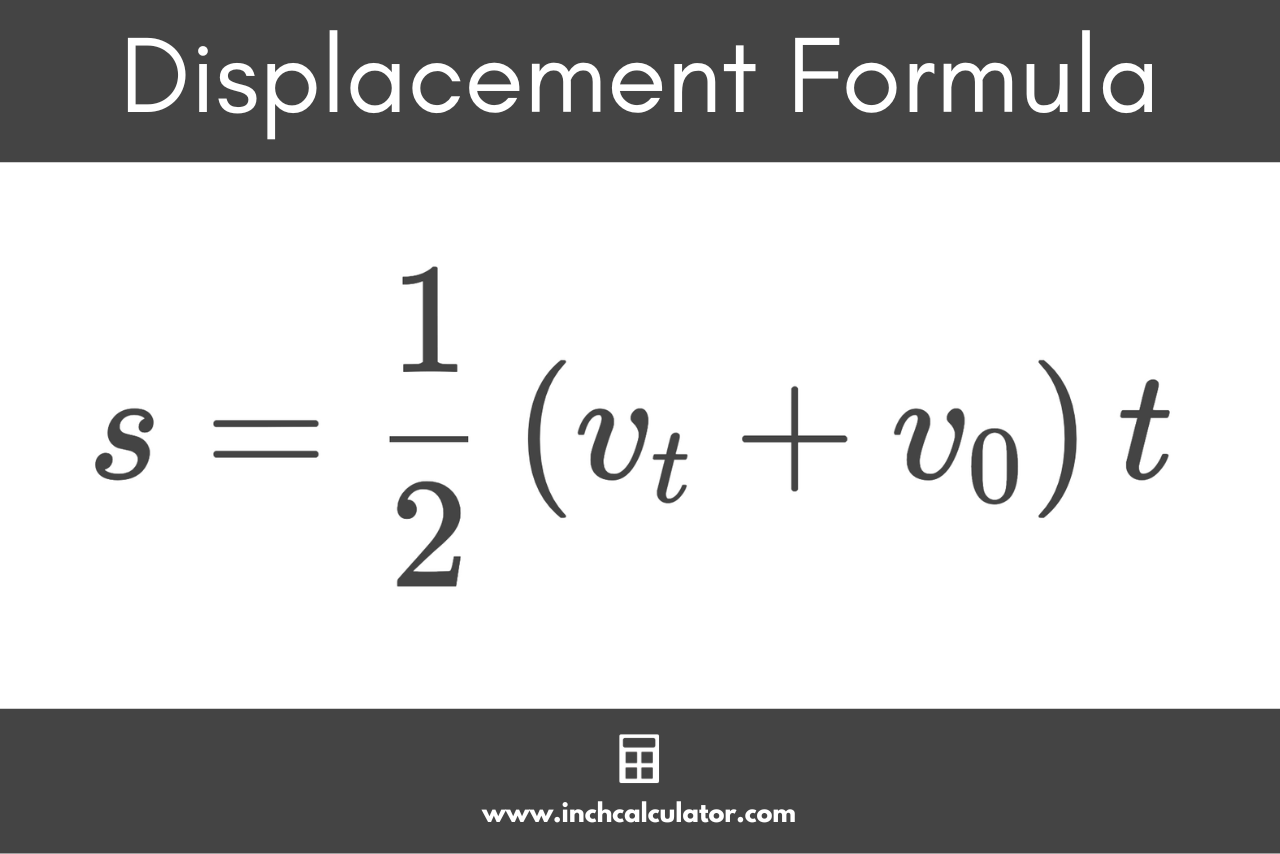# Displacement Calculator

Use our displacement calculator to find the displacement, initial velocity, final velocity, or duration of time.

Calculate:

## Displacement:

-

### Displacement Formula

s=\frac{1}{2} \left ( v_{t}+v_{0} \right ) t
Learn how we calculated this below

## How to Calculate Displacement

Displacement, in physics, refers to the overall change in the position of an object. It is a vector quantity, meaning it has both magnitude and direction.

You can calculate displacement using a simple formula.

### Displacement Formula

The formula for displacement s can be expressed as the difference between the final position x2 minus and the initial position x1.

s=x_{2}−x_{1}

If you know the velocity of a moving object, then you can also calculate displacement using the following formula:

s=\frac{1}{2} \left ( v_{t}+v_{0} \right ) t

Thus, the displacement s is equal to the average velocity times the time duration t. The average velocity can be found by subtracting the initial velocity v0 from the final velocity vt, then dividing the result in half.## Difference Between Displacement and Distance

It’s important to note that the displacement is not always equal to the total path length traveled. Instead, it is the shortest distance between the initial and final positions, and it takes into consideration the direction of motion.

Displacement, as mentioned above, considers the starting and ending points of motion and is a vector.

Distance, on the other hand, is a scalar quantity, which means it only concerns the magnitude, not direction. Distance is the total path length traveled by an object, regardless of the direction.

Thus, while the distance can never be negative, displacement can, indicating a change in the opposite direction.

For example, if you walked 5 meters forward and then 5 meters backward, your total distance would be 10 meters, but your displacement would be 0 meters.